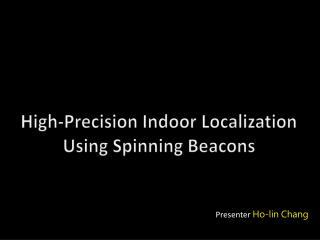DownloadDownload PresentationHigh-Precision Indoor Localization Using Spinning Beacons

High-Precision Indoor Localization Using Spinning Beacons

Télécharger la présentationHigh-Precision Indoor Localization Using Spinning Beacons

- - - - - - - - - - - - - - - - - - - - - - - - - - - E N D - - - - - - - - - - - - - - - - - - - - - - - - - - -
Presentation Transcript

1. High-Precision Indoor Localization Using Spinning Beacons Presenter Ho-lin Chang

2. Outline Introduction Design Implementation Evaluation Conclusion and future Work

3. Introduction- Motivation Warehouse Security Healthcare Indoor location-based service

4. Introduction – Related work WiFi UWB Ultrasound • Existed indoor localization technique • UWB • Ultrasound • WiFi

5. Introduction – Related work • UWB (Ubisense) • Accuracy: 10 ~ 20 cm • Time difference of arrival • Expensive specialized hardware (10,000 USD)

6. Introduction – Related work • Ultrasonic (Cricket) • Accuracy: 10 ~ 20 cm • Short range • Non-line of sight problem

7. Introduction – Related work • WiFi (Ekahau, RADAR) • RSS fingerprinting • Accuracy: 3 ~ 5m • Low cost • Offline training

8. Introduction– Indoor localization

9. Introduction – Contribution • Develop a novel localization system • Spinning beacon (RF) • Indoor environment • Sub-meter accuracy • 50%< 39 cm • 90%< 70 cm • Low cost • Low cost motes (100 USD) • Rotation motor

10. Design

11. Doppler Angulation Doppler Angulation 2nd Doppler Angulation 3rd Doppler Angulation S S X S X R Localization The location of X

12. Design – Doppler Effect X f S vproject v

13. Design – When the beacon spins … Δf = 0 Hz Δf = -30 Hz Δf = 30 Hz Δf = 0 Hz X -30Hz 0Hz 0Hz v S 30Hz frequency Δf (t) = ? time

14. Design – Model the frequency waveform v(t) = (-ωr sinθ, ωr cosθ) y S : (r cosθ, r sinθ) X : (d cosα, d sinα) X d α r v(t) θ(t) = ωt+φ x S

15. Design – Doppler angulation y X α v(t) R β x S

16. System overview

17. Doppler Angulation Frequency observation 2nd Doppler Angulation S S 3rd Doppler Angulation X S X Time delay estimation R Localization centroid The location of X

18. Typical RF frequency is too high. • Radio Interferometry • “Radio InterferometricGeolocation” • [sensys ‘05] Doppler Angulation Frequency observation 2nd Doppler Angulation 3rd Doppler Angulation Time delay estimation 900 MHz B A 900 MHz + 500 Hz Localization centroid 500 Hz The location of X

19. Delay-and-compare method Doppler Angulation Frequency observation 2nd Doppler Angulation 3rd Doppler Angulation Time delay estimation Localization centroid The location of X

20. Implementation • Software • TinyOS 1.x • C/C++ • Hardware • Crossbow MICA2 • Rotational motor

21. Evaluation • Testbed • 國發所地下室停車場 • Three spinning beacons • 30 sample points (2m grid) • 10 position samples (300 samples) • 3 angles (900 angles) • Evaluation metrics • Positional error • Angular error • ParameterTuning

22. Evaluation – Angular accuracy 90% < 10 degrees 90% < 10 degrees 50% < 3 degrees 50% < 3 degrees

23. Evaluation – Positional accuracy 90% < 70cm 90% < 10 degrees 50% < 3 degrees 50% < 39cm

24. Evaluation – Parameter tuning • Data collection time • Rotational velocity • Interference frequency • Angulation filtering threshold • Minimumdistanceasaqualityindicator

25. Evaluation– Data collection time

26. Evaluation – Rotational velocity

27. Evaluation – Interference frequency Lower frequency estimation precision

28. Evaluation– Filtering threshold

29. System Limitation Track fast moving targets Rotational device

30. Conclusion • Develop a novel localization system • Spinning beacon • Indoor environment • Low cost • Sub-meter accuracy • 39cm / 70cm

31. Future Work • Reduce the localization latency • Reduce the routing time • Distributed version • Data compression • Track the fast moving targets

32. Q&A

33. Thanks

34. Design – Approximation r/d 0 0.1 0.3 0.5

35. Frequency waveform approximation

36. Radio interferometry fA |fA + ΔfX - fB| |fA-fB| v X A B fB

37. Real Deployment S S A S R

38. Signal strength Time

39. FDOA (Frequency Difference of Arrival) I I I v X I I I • Each infrastructure perceives different Doppler shift. • Localize the target by different Doppler shifts

40. Maximum Doppler shift • f : 900 MHz • ω :2.5round/sec • r : 30 cm ~ 50 cm

41. Localization latency 0.3 ~ 1.5sec 8 ~ 10 sec 1st Doppler angulation Data collection Routing 2nd Doppler angulation Data collection Routing 3rd Doppler angulation Data collection Routing Localization Localization

42. Design – Model the frequency waveform v(t) = (-ωr sinθ, ωr cosθ, 0) y S : (r cosθ, r sinθ, 0) X : (d cosα, d sinα, h) X d α r v(t) θ(t) = ωt+φ x S